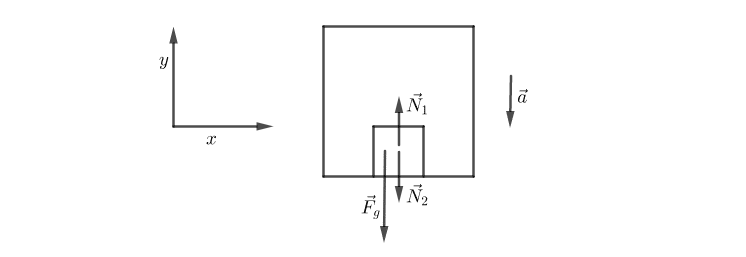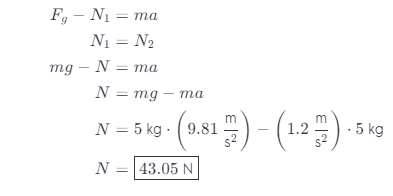# A 5.0 Kg Dog Sits On The Floor Of An Elevator That Is Accelerating Downward At 1.20 M/s2.

We thoroughly check each answer to a question to provide you with the most correct answers. Found a mistake? Let us know about it through the REPORT button at the bottom of the page.

A 5.0 kg dog sits on the floor of an elevator that is accelerating downward at 1.20m/s^2.

a. What is the magnitude of the normal force of the elevator floor on the dog?

A. 34 N

B. 43 N

C. 49 N

D. 55 N

E. 74 N

Contents

A) B

B) D

## Explanation

Given values:

m = 5 kg

a = 1.20 m/s^2

Let us sketch the lines of forces we have:The force of the normal reaction of the substrate acting on the dog N1 will be equal to the normal force exerted by the dog on the substrate N2, according to Newton’s third law of action and reaction.

According to second Newton’s second law, we have:

F = ma

N1 – Fg = -ma

Minus sign because the y-axis is directed opposite to the direction of movement of the elevator.